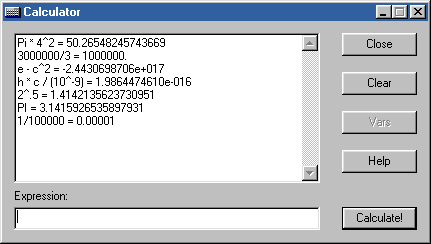[an error occurred while processing this directive] Calculator

CalculatorCurrent version: 3.25.Download Calculator.exe

Required files: [MFC42D.DLL] [MSVCRTD.DLL] [MFCO42D.DLL]

Help
The calculator evaluates mathematical expressions. It converts them from conventional form to a postfix string, which is later evaluates. Examples of input: 1+1 will render 2. 1+2*3 is 7.
The calculator can:
• Perform simple algebraic operations: +, -, /, *, : (same as /), ^ (to the power of), % (modulo), parentheses, and decimals. ~ denotes negative.
• Like TI®, if the expression begins with an operator, the answer is assumed as the first operand. E.g. If you type "1+1", and then "+1", then the answer is going to be 3.
• a is the answer of previous calculation. Default is 0.
• Constants include: PI, e, R (ideal gas constant), g (acceleration due to gravity, negative), h (Planck's constant), and c (the speed of light). They may be used in place of numbers. Capitalization does not matter.
• Current functions include abs, acos, arccos, asin, arcsin, atan, arctan, cos, cosh, gcd, int, lcm, ln, log, pow, rand, rnd, sin, sinh, sqrt, tan, and tanh! Format: "sin (pi / 2)", "sin 5"; "gcd (12, 100)"; "lcm (50, 60)".
• You can choose the randomness of numbers: C++ style (a number, such as 19773), or BASIC style (0.1954952)
• Pick either degrees or radians.
• I have not found a way to crash it. It is (supposedly) fool-proof. :-)
Notes:
• Both ~ and - can be used to negate a number, only when the expression begins with -, a-... is assumed.
• Contrary to popular belief, precedence of power operator is from right to left.
• Program performs as well as the processor allows it. Decimals may be inacurate. e.g. 10^9+3*10^-7 = 1000000000.0000004
• Negative numbers to the fraction powers, such as 1/2 and 1/4, equal either 0 or nan (not a number).
• Alt+F4 does not work: both this and <Esc> clear the history.
• Gcd and lcd of a single operand will return that single operand.
• To implement gcm and lcd, I had to use a stack of doubleints (a struct of two ints), and a queue of ints. When a gcd parenthesis is closed, its number of parameters (counted by number of commas) is enqueued. A stack is used for nested gcds and lcms: you can say, "gcd (15, 20, lcm (7, 5))". It took me about 5 hours to implement this. :-)
• Capitalization does not matter.
• Pow takes only two parameters.
• Double-clicking on either a function on a constant closes the option dialog and enters the entry into the expression string.
• For coding purposes, rand is defined as a constant. :-)

Click on the button to see description

 Calculator
 100+100 = 200. Note: I am not a working model. :-) Expression:

Version History

3.25 New! Improvements:
• Options window works! Two options: randomness (BASIC: a real number 0 to 1; and C++: something like 31758), and trig (radians or degrees).
• A complete listing of all the functions and constants in Options window. Double-clicking will append the selected value to the expression.
• Arcsin, arccos, and pow functions added!
3.1 Improvements:
• They are here! Functions! Sin, cos, and so many more! (Current functions include abs, acos, arccos, asin, arcsin, atan, arctan, cos, cosh, gcd, int, lcm, ln, log, pow, rand, rnd, sin, sinh, sqrt, tan, and tanh!)
• The two multi-parameter functions are lcm and gcd. Format: lcd(12, 16, 20). Comma is used as a separator.
• Factorial bug fix. Now, 3.5! does not equal to 12.125.
• A few changes in error wordings...
3.0 Fixes of 2.5. New Options:
• Included: constants. These include PI, e, c, h, R, and g.
• To be added: a Variable & Constant window, which will display all constants.

2.5 Improvements over 2.0:
• Improved exception handling.
• New operator: ":", which is the same as "/".
• Fixed the output, up to 14 decimal places.
• History box scrolls automatically.
• Minor code fixes

2.0 Restarted from Postfix.cpp.
• Made a window that handles the input and output; everything is Windows-based.
• Rewrote the code to be transferrable: exceptions handle all errors.
• Fixed loads of exception and other yucky errors.
• Made to accept rational numbers

1.0 The first program.
• Performs most algebraic expressions.
• Accepts multidigit integers.
• Knows the order of operations.
• Knows parenthesis.

Known Bugs:
• Cannot control imaginary numbers
• Floating point operations vary with platforms: computer cannot handle, say, 10^9+10^-7 perfectly.
• Anything else? Contact me.

Back

[an error occurred while processing this directive]